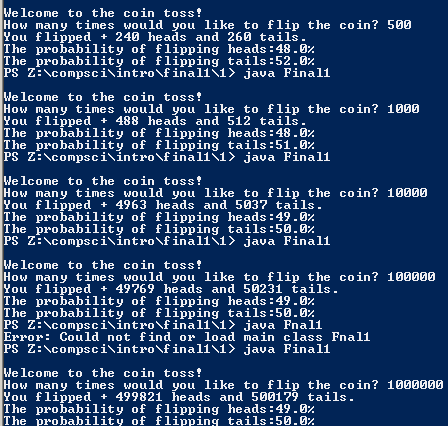# Final1

## Code

```    /// Name: Ian Stanko
/// Period: 5
/// Program Name: Final1
/// File Name: Final1.java
/// Date Finished: 1/20/2016

import java.util.Scanner;
import java.util.Random;

public class Final1
{

public static void main(String[] args)
{

Scanner keyboard = new Scanner(System.in);
Random r = new Random();

int flips = 1, heads = 0, tails = 0;

System.out.println( "Welcome to the coin toss!" );
System.out.print( "How many times would you like to flip the coin? " );
flips = keyboard.nextInt();

for ( int i = 1; i <= flips; i++ )
{
int side = 1;
side = r.nextInt(2);

if ( side == 0 )
{
} else
{
tails++;
}
}

double probOfTails = 100 * tails / flips;

System.out.println( "You flipped + " + heads + " heads and " + tails + " tails." );
System.out.println( "The probability of flipping tails:" + probOfTails + "%" );

}
}

///i used a loop because it repeated the flip as many times as was input
///if statements were used because it allows to add up the number of heads or tails to find the probability in the end
///around 10000 flips the probabilities start to level off at 50% and 49% but dont seem to get closer to 50%-50% the larger you make the number of flips

```

### Picture of the output HOME